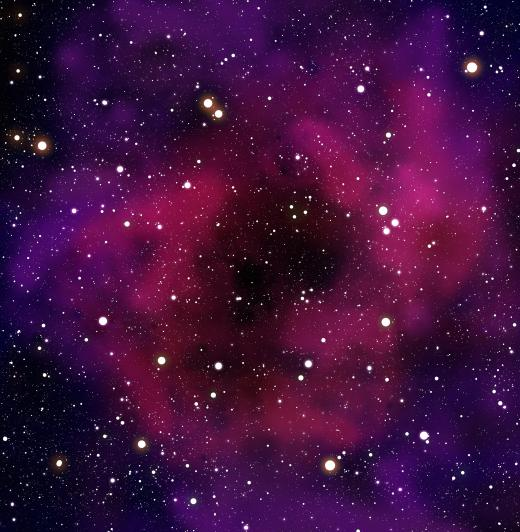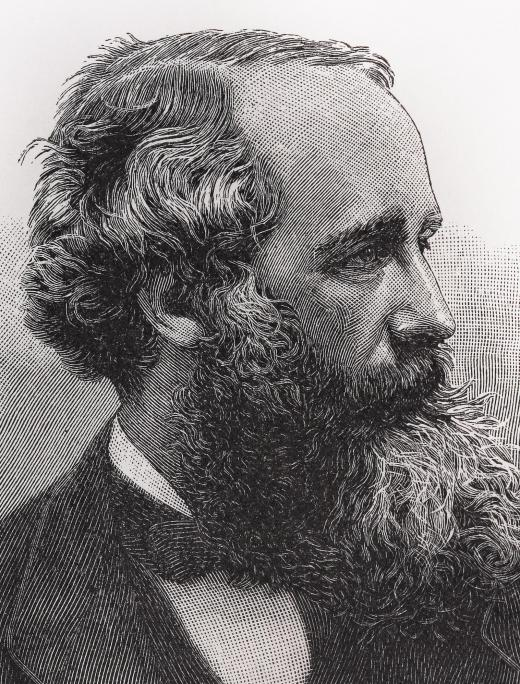Science
Fact Checked

# What Is a Grand Unified Theory?

Ray Hawk
Ray Hawk

The Grand Unified Theory (GUT) is the search for a concise, simple theory in physics as of 2011 that would describe the interaction between the four fundamental forces of nature — electromagnetism, the weak and strong nuclear forces, and gravity. Since any grand unified theory attempts to bring together current understanding of physical forces, it is often referred to as research in classical unified field theories. Many different unified field theories exist to explain the behavior of matter and energy, yet string theory is seen as the best prospect as of 2011 for having a chance of tying the effects of gravity to the other three fundamental forces.

Particle physics currently uses a theory known as the Standard Model to describe the interaction of physical forces in nature. The Standard Model's current form as of 2011 was established in the 1970s and accounts for the interaction of three of the four fundamental forces — electromagnetism and the weak and strong nuclear forces. As the Standard Model has developed, it was revealed that electromagnetism and the weak nuclear force were really aspects of the same force at high levels of energy. This built upon earlier discoveries in the late-19th century by the British physicist James Maxwell, uniting the forces of magnetism and electricity as being two aspects of the same force — electromagnetism. The Grand Unified Theory, therefore, can be considered to be an attempt to unite the Standard Model with the effects of gravity.The Grand Unified Theory is an attempt to simplify man's understanding of all forces of nature.

Since the development of a simple grand unified theory might not account for all interactions of matter and energy in space, often the final theory unifying all forces is referred to as the Theory of Everything (TOE). Three main arenas of study in physics would have to be united into one to produce a viable theory of everything, and these include the Standard Model, general relativity, and quantum mechanics. One key proposal for this involves research into string theory, which describes a fundamental state of matter where everything in the physical universe is ultimately composed of vibrating loops of energy, each the size of a Planck length, or 10-33 centimeters. These strings are theorized to be the direct cause for the effects of all four fundamental forces in nature, the existence of matter and energy, and both space and time as well. One of the weaknesses of string theory, however, is that it is extremely complex, and requires interactions in ten dimensions of space, whereas an ultimate grand unified theory is envisioned as being a simple equation no longer than about 1 inch (2.5 centimeters) in length if written out at the usual size of handwriting.Electromagnetism, which was studied by James Clerk Maxwell, is one of the four fundamental forces of nature.

Perhaps the greatest challenge to coming up with an elegant form of a grand unified theory as of 2011, however, is that of working the physical cosmology of gravity into the equations. Gravity is the least understood of all physical forces, and may not be a force at all. Some research suggests that gravity is simply an effect of the warping of space by matter as Einstein's discoveries suggest, because an origin for the source of gravity has never been found. All other forces in nature have fundamental particles tied to them, so physics has assumed a graviton particle would exist to explain gravity. Until evidence is obtained to explain how gravity works at both a macroscopic and microscopic level, a Grand Unified Theory uniting all physical forces in nature will remain elusive.

## You might also Like